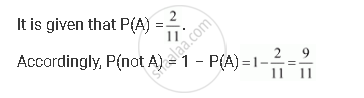CBSE (Arts) Class 11CBSE
Share

# If 2/11 is the Probability of an Event, What is the Probability of the Event ‘Not A’. - CBSE (Arts) Class 11 - Mathematics

ConceptProbability Probability of 'Not', 'And' and 'Or' Events

#### Question

If 2/11 is the probability of an event, what is the probability of the event ‘not A’.

#### SolutionIs there an error in this question or solution?

#### APPEARS IN

Solution If 2/11 is the Probability of an Event, What is the Probability of the Event ‘Not A’. Concept: Probability - Probability of 'Not', 'And' and 'Or' Events.
S